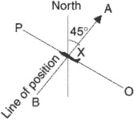# Optical Path

## optical path

[′äp·tə·kəl ′path]
(optics)
For a ray of light traveling along a path between two points, the optical path is the integral, over elements of length along the path, of the refractive index. Also known as optical distance; optical length.

## Optical Path

The optical path between points A and B of a transparent medium is the distance optical radiation, or light, would travel in a vacuum during the time it takes to move from A to B. Since the speed of light in any medium is less than in a vacuum, the optical path is always greater than the distance actually traversed by the light except in the limiting case of a vacuum, where the optical path is equal to the distance traversed.

The trajectory of a light beam in an optical system consisting of ρ homogeneous media is a broken line. In such a system the optical path is equal to [com] Σp/knk, where lk is the distance traveled by the light in the kth medium (k = 1,2,…,p),nk is the refractive index of the medium, and Σ is the summation sign. For one medium (p = 1), the sum is reduced to a single term ln. In an optically inhomogeneous medium with a smoothly changing n, the trajectory of a beam is a curve. The optical path in such a medium is [com] ∫ABn(l) dl, where dl is an infinitely small element of the beam trajectory.

The concept of optical path plays a major role in optics, especially geometrical and crystal optics, because it makes possible comparison of the paths traversed by light in media in which the rate of propagation differs. The locus of points for which the optical paths, reckoned from the same source, are identical is called the surface of the light wave; light oscillations on this surface are in the same phase.

### REFERENCES

Landsberg, G. S. Optika, 4th ed. Moscow, 1957. (Obshchii kurs fiziki, vol. 3.)
Tudorovskii, A. I. Teoriia opticheskikh priborov, 2nd ed., part 1. Moscow-Leningrad, 1948.
Born, M, and E. Wolf. Osnovy optiki, 2nd ed. Moscow, 1973. (Translated from English.)

## line of position (LOP)Aircraft flying from O to P obtains a bearing of 045° from A. Its position lies anywhere along the line AB.
In air navigation, a line along which an aircraft is known to be traveling and which may be utilized to establish a position or a fix. This line can be straight or curved, depending on the source of information: a true bearing from a mountain peak will be a straight line of positions; the distance from an object will be a circle of radius equal to that distance. Two or more LOPs are required to establish a fix.
line of sight
i. The straight line between two points. This line is in the plane of the great circle but does not follow the contour of the earth. Radar and some forms of radio waves are capable of being received in the line of sight.
ii. The straight line between the eye of an observer and the observed object or point. Also called an optical path.
iii. In radio, a direct propagation path that does not go below the radio horizon.
References in periodicals archive ?
One is the conservation rule of energy, and the other is equal optical path. The formal and the later rules dedicate the amplitude and the phase distribution, respectively.
The ratio of the therapeutic to erythema radiation has a minimum value at solar noon (diurnal minimum optical path length).
30 April 2012 a[euro]" US measurement solutions provider Agilent Technologies Inc (NYSE:A) has entered into a definitive agreement to acquire the test and measurement unit of Centellax, a California-based supplier of high-performance test instruments and components for electrical and optical path signal integrity testing.
According to the coupling theory, when the two output arms of the optical path are different, there is an optical path difference nd (n is the refractive index of fiber), the phase difference corresponding is
A redesigned optical path includes an increased number of elements and two aspherical lenses for fast, accurate performance, whilst Canon's acclaimed Optical Image Stabilizer (OIS) technology provides distortion-free images throughout the range of the 10x optical zoom.
Atrium Network's ultra-low latency Exchange Ring is connected to PLUS-SX via Atrium Network's point of presence in PLUS-SX's data centre, allowing PLUS-SX to take advantage of a low-latency direct optical path and consolidated data feeds offered by Atrium.
The laser with frequency [f.sub.o] is emitted and divided with a half-mirror (HM) into the sensing optical path and detecting optical path.
The optical path is long and this introduces errors.
The OBM group has embarked on the building of a proof-of-concept demonstration which will deliver a volumetric monochrome image 80 mm wide by 60 mm high and 80 mm deep, of 440 scan lines at 30 Hz frequency, with a 24[degrees] angle of view, all with an optical path length approx 1500 mm so that the whole system will fit into a shallow box.
By actuating the optical path internal to the instrument until the two path lengths are made equal and an interferometric signal is detected, the optical path of the sensor can be measured.
Light is deviated along the optical path from its nominal course in the absence of refractive--index variations.
The parallel optical path created by the Infinity objectives allows for the addition of components below the head, such as the epi-fluorescent or polarizing system for specialized applications.

Site: Follow: Share:
Open / Close